Over a million developers have joined DZone.{{announcement.body}}
{{announcement.title}}

DZone's Guide to

# Classification From Scratch, Part 2 of 8: Logistics With Splines

In Part 2 of our series on data regression, we take a look at different types of splines (linear, quadratic, and cubic) and how to represent them with functions in R.

· Big Data Zone ·
Free Resource

Comment (0)

Save
{{ articles.views | formatCount}} Views

Cloudera Data Flow, the answer to all your real-time streaming data problems. Manage your data from edge to enterprise with a no-code approach to developing sophisticated streaming applications easily. Learn more today.

Today, we dive into the second post of our series on classification from scratch, following the brief introduction to logistic regression.

## Piecewise Linear Splines

To illustrate what's going on, let us start with a "simple" regression (with only one explanatory variable). The underlying idea is natura non facit saltus, for "nature does not make jumps," i.e. the processes governing equations for natural things are continuous. That seems to be a rather strong assumption, because we can assume that there is a fixed threshold to explain death. For instance, if patients die (for sure) if the "stroke index" exceeds a threshold, we might expect some discontinuity. Except that if that threshold is a heterogeneous (non-observable continuous) variable, then we get back to the continuity assumption.

The simplest model we can think of to extend the linear model we've seen in the previous post is to consider a piecewise linear function with two parts: small values of x, and larger values of x. The most convenient way to do so is to use the positive part function (x-s)+, which is the difference between x and s if that difference is positive, and 0 otherwise. For instance: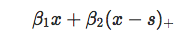is the following piecewise linear function, continuous, with a "rupture" at knot s.Observe also the following interpretation: for small values of x, there is a linear increase, with slope ß1, and for larger values of x, there is a linear decrease, with slope ß1 + ß2. Hence, ß2 is interpreted as a change of the slope.

And, of course, it is possible to consider more than one knot. The function to get the positive values is the following:

``pos = function(x,s) (x-s)*(x&gt;=s)``

Then we can use it directly in our regression model:

``````reg = glm(PRONO~INSYS+pos(INSYS,15)+
pos(INSYS,25),data=myocarde,family=binomial)``````

The output of the regression is here:

``````summary(reg)

Coefficients:
Estimate Std. Error z value Pr(&gt;|z|)
(Intercept)     -0.1109     3.2783  -0.034   0.9730
INSYS           -0.1751     0.2526  -0.693   0.4883
pos(INSYS, 15)   0.7900     0.3745   2.109   0.0349 *
pos(INSYS, 25)  -0.5797     0.2903  -1.997   0.0458 *``````

Hence, the original slope for very small values is not significant, but then, above 15, it becomes significantly positive. And above 25 there is a significant change again. We can plot it to see what's going on:

``````u = seq(5,55,length=201)
v = predict(reg,newdata=data.frame(INSYS=u),type="response")
plot(u,v,type="l")
points(myocarde\$INSYS,myocarde\$PRONO,pch=19)
abline(v=c(5,15,25,55),lty=2)``````## Using bs() Linear Splines

Using the GAM function, things are slightly different. Here, we will use the so-called b-splines:

``library(splines)``

We can define spline functions with support (5,55) and with knots {15,25}:

``````clr6 = c("#1b9e77","#d95f02","#7570b3","#e7298a","#66a61e","#e6ab02")
x = seq(0,60,by=.25)
B = bs(x,knots=c(15,25),Boundary.knots=c(5,55),degre=1)
matplot(x,B,type="l",lty=1,lwd=2,col=clr6)``````As we can see, the functions defined here are different from the one before, but we still have (piecewise) linear functions on each segment (5,15), (15,25) and (25,55). But linear combinations of those functions (the two sets of functions) will generate the same space. Said differently, if the interpretation of the output are different, the predictions should be the same:

``````reg = glm(PRONO~bs(INSYS,knots=c(15,25),
Boundary.knots=c(5,55),degre=1),
data=myocarde,family=binomial)
summary(reg)

Coefficients:
Estimate Std. Error z value Pr(&gt;|z|)
(Intercept)    -0.9863     2.0555  -0.480   0.6314
bs(INSYS,..)1  -1.7507     2.5262  -0.693   0.4883
bs(INSYS,..)2   4.3989     2.0619   2.133   0.0329 *
bs(INSYS,..)3   5.4572     5.4146   1.008   0.3135``````

Observe that there are three coefficients, as before, but again, the interpretation is here more complicated...

``````v=predict(reg,newdata=data.frame(INSYS=u),type="response")
plot(u,v,ylim=0:1,type="l",col="red")
points(myocarde\$INSYS,myocarde\$PRONO,pch=19)
abline(v=c(5,15,25,55),lty=2)``````Nevertheless, the prediction is the same... and that's nice.

## Piecewise Quadratic Splines

Let us go one step further... Can we have also the continuity of the derivative ? Yes, and that's easy actually, considering parabolic functions. Instead of using a decomposition on x, (x-s1)+ and (x-s2)+ consider now a decomposition on x ,x2,(x-s1)2+ and (x-s2)2+.

``````pos2 = function(x,s) (x-s)^2*(x&gt;=s)
reg = glm(PRONO~poly(INSYS,2)+pos2(INSYS,15)+pos2(INSYS,25),
data=myocarde,family=binomial)
summary(reg)

Coefficients:
Estimate Std. Error z value Pr(&gt;|z|)
(Intercept)      29.9842    15.2368   1.968   0.0491 *
poly(INSYS, 2)1 408.7851   202.4194   2.019   0.0434 *
poly(INSYS, 2)2 199.1628   101.5892   1.960   0.0499 *
pos2(INSYS, 15)  -0.2281     0.1264  -1.805   0.0712 .
pos2(INSYS, 25)   0.0439     0.0805   0.545   0.5855``````

As expected, there are five coefficients here: the intercept and two for the part on the left (three parameters for the parabolic function), and then two additional terms for the part in the center - here (15,25) - and for the part on the right. Of course, for each portion, there is only one degree of freedom since we have a parabolic function (three coefficients) but two constraints (continuity, and continuity of the first order derivative).

On a graph, we get the following:

``````v = predict(reg,newdata=data.frame(INSYS=u),type="response")
plot(u,v,ylim=0:1,type="l",col="red",lwd=2,xlab="INSYS",ylab="")
points(myocarde\$INSYS,myocarde\$PRONO,pch=19)
abline(v=c(5,15,25,55),lty=2)``````## Using bs() Quadratic Splines

Of course, we can do the same with our R function. But as before, the basis of the function is expressed differently here:

``````x = seq(0,60,by=.25)
B=bs(x,knots=c(15,25),Boundary.knots=c(5,55),degre=2)
matplot(x,B,type="l",xlab="INSYS",col=clr6)``````If we run R code, we get:

``````reg = glm(PRONO~bs(INSYS,knots=c(15,25),
Boundary.knots=c(5,55),degre=2),data=myocarde,
family=binomial)
summary(reg)

Coefficients:
Estimate Std. Error z value Pr(&gt;|z|)
(Intercept)       7.186      5.261   1.366   0.1720
bs(INSYS, ..)1  -14.656      7.923  -1.850   0.0643 .
bs(INSYS, ..)2   -5.692      4.638  -1.227   0.2198
bs(INSYS, ..)3   -2.454      8.780  -0.279   0.7799
bs(INSYS, ..)4    6.429     41.675   0.154   0.8774``````

But that's not really a big deal since the prediction is exactly the same:

``````v = predict(reg,newdata=data.frame(INSYS=u),type="response")
plot(u,v,ylim=0:1,type="l",col="red")
points(myocarde\$INSYS,myocarde\$PRONO,pch=19)
abline(v=c(5,15,25,55),lty=2)``````## Cubic splines

Last, but not least, we can reach the cubic splines. With our previous notions, we would consider a decomposition on (guess what) x, x2, x3, (x-s1)3+, (x-s2)3+, to get this time continuity, as well as continuity of the first two derivatives (and to get a very smooth function, since even variations will be smooth). If we use the bs function, the basis is the following:

``````B=bs(x,knots=c(15,25),Boundary.knots=c(5,55),degre=3)
matplot(x,B,type="l",lwd=2,col=clr6,lty=1,ylim=c(-.2,1.2))
abline(v=c(5,15,25,55),lty=2)``````

And the prediction will now be:

``````reg = glm(PRONO~bs(INSYS,knots=c(15,25),
Boundary.knots=c(5,55),degre=3),
data=myocarde,family=binomial)
u = seq(5,55,length=201)
v = predict(reg,newdata=data.frame(INSYS=u),type="response")
plot(u,v,ylim=0:1,type="l",col="red",lwd=2)
points(myocarde\$INSYS,myocarde\$PRONO,pch=19)
abline(v=c(5,15,25,55),lty=2)``````Just two more things before concluding (for today): the location of the knots and the extension to additive models.

## Location of Knots

In many applications, we do not want to specify the location of the knots. We just want - say - three (intermediary) knots. This can be done using:

``reg = glm(PRONO~1+bs(INSYS,degree=1,df=4),data=myocarde,family=binomial)``

We can actually get the locations of the knots by looking at:

``````attr(reg\$terms, "predvars")[]
bs(INSYS, degree = 1L, knots = c(15.8, 21.4, 27.15),
Boundary.knots = c(8.7, 54), intercept = FALSE)``````

This provides us with the location of the boundary knots (the minimum and the maximum from our sample) but also the three intermediary knots. Observe that, actually, those five values are just (empirical) quantiles:

``````quantile(myocarde\$INSYS,(0:4)/4)
0%   25%   50%   75%  100%
8.70 15.80 21.40 27.15 54.00``````

If we plot the prediction, we get:

``````v = predict(reg,newdata=data.frame(INSYS=u),type="response")
plot(u,v,ylim=0:1,type="l",col="red",lwd=2)
points(myocarde\$INSYS,myocarde\$PRONO,pch=19)
abline(v=quantile(myocarde\$INSYS,(0:4)/4),lty=2)``````If we get back on what was computed before the logit transformation, we clearly see ruptures are the different quantiles:

``````B = bs(x,degree=1,df=4)
B = cbind(1,B)
y = B%*%coefficients(reg)
plot(x,y,type="l",col="red",lwd=2)
abline(v=quantile(myocarde\$INSYS,(0:4)/4),lty=2)``````Note that if we do specify anything about knots (number or location), we get no knots...

``````reg = glm(PRONO~1+bs(INSYS,degree=2),data=myocarde,family=binomial)
attr(reg\$terms, "predvars")[]
bs(INSYS, degree = 2L, knots = numeric(0),
Boundary.knots = c(8.7,54), intercept = FALSE)``````

and if we look at the prediction

``````u = seq(5,55,length=201)
v = predict(reg,newdata=data.frame(INSYS=u),type="response")
plot(u,v,ylim=0:1,type="l",col="red",lwd=2)
points(myocarde\$INSYS,myocarde\$PRONO,pch=19)``````Actually, it is the same as a quadratic regression (as expected actually):

``````reg = glm(PRONO~1+poly(INSYS,degree=2),data=myocarde,family=binomial)
v = predict(reg,newdata=data.frame(INSYS=u),type="response")
plot(u,v,ylim=0:1,type="l",col="red",lwd=2)
points(myocarde\$INSYS,myocarde\$PRONO,pch=19)``````Consider now the second dataset, with two variables. Consider here a model like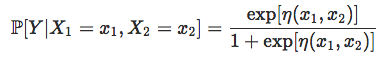where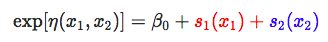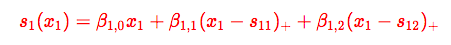and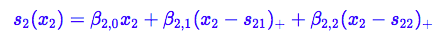It might seem a little bit restrictive, but that's actually the idea of additive models.

``````reg = glm(y~bs(x1,degree=1,df=3)+bs(x2,degree=1,df=3),data=df,family=binomial(link = "logit"))
u = seq(0,1,length=101)
p = function(x,y) predict.glm(reg,newdata=data.frame(x1=x,x2=y),type="response")
v = outer(u,u,p)
image(u,u,v,xlab="Variable 1",ylab="Variable 2",col=clr10,breaks=(0:10)/10)
points(df\$x1,df\$x2,pch=19,cex=1.5,col="white")
points(df\$x1,df\$x2,pch=c(1,19)[1+(df\$y=="1")],cex=1.5)Now, if we think about it, we've been able to get a "perfect" model, so, somehow, it no longer seems continuous...Of course, it is... it is piecewise linear, with hyperplane, some being almost vertical.

And one can also consider piecewise quadratic functions:

``````reg = glm(y~bs(x1,degree=2,df=3)+bs(x2,degree=2,df=3),data=df,family=binomial(link = "logit"))
u = seq(0,1,length=101)
p = function(x,y) predict.glm(reg,newdata=data.frame(x1=x,x2=y),type="response")
v = outer(u,u,p)
image(u,u,v,xlab="Variable 1",ylab="Variable 2",col=clr10,breaks=(0:10)/10)
points(df\$x1,df\$x2,pch=19,cex=1.5,col="white")
points(df\$x1,df\$x2,pch=c(1,19)[1+(df\$y=="1")],cex=1.5)Funny thing, we now have two "perfect" models, with different areas for the white and the black dots... Don't ask me how to choose on that one.

In R, it is possible to use the mgcv package to run a gam regression. It is used for generalized additive models, but here, we have only one variable, so it is difficult to see the "additive" part, actually. And to be more specific, mgcv is using penalized quasi-likelihood from the nlme package (but we'll get back on penalized routines later on).

But maybe I should also mention another smoothing tool before kernels (and maybe also k-nearest neighbors). To be continued...

Cloudera Enterprise Data Hub. One platform, many applications. Start today.

Topics:
big data ,r ,regression models ,splines ,predictive analytics

Comment (0)

Save
{{ articles.views | formatCount}} Views

Published at DZone with permission of

Opinions expressed by DZone contributors are their own.

# {{ parent.title || parent.header.title}}

{{ parent.tldr }}

{{ parent.urlSource.name }}